# Correcting Errors

Who might have realized that when the mathematics community studied the properties of cubes, they might be used in error correction technology?

# Correcting Errors

Joe Malkevitch
York College (CUNY)

## Introduction

Humans sometimes make errors. Perhaps you have been sloppy with a calculation on a mathematics examination. You made an error. While many see machines and communications systems as more reliable than humans when it comes to making errors, machines and communications systems do make errors too. One can think of this as the consequence of there being noise in any physical communication system, which by chance alters the information involved. Advances in sending information electronically, including streaming sound and video, have led mathematicians to take an interest in increasing the reliability of data which is transferred electronically. Digital technologies work so well that we often now have lost track of the mathematical triumphs that make these technologies as reliable as they are. An earlier column looked at some aspects of using codes to correct errors in digital technology systems. Here I will look at a kind of error that communications systems are subject to, errors which arise due to a symbol in a code word being dropped (or inserted) rather than changed. Deletion errors have recently gotten renewed attention and are much less understood than other kinds of transmission errors. First, let me provide some background related to using mathematics to correct errors.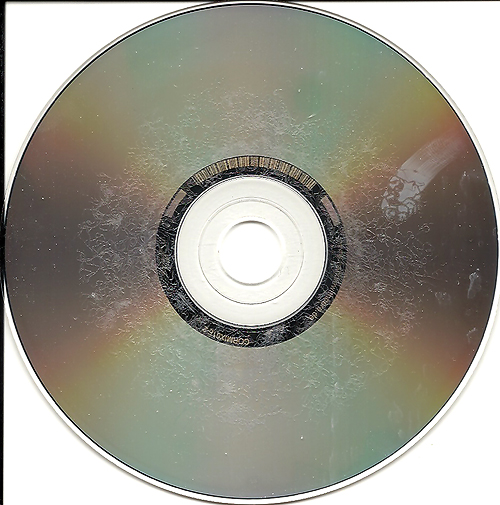Can the data on a damaged disc still be read? (Image by Nathanael Coyne, CC BY-NC-ND 2.0)

## Basic ideas

Mathematics is often viewed as studying properties of patterns involving numbers or shapes, the investigations that give rise to the areas of mathematics called algebra and geometry (topology). We are familiar with numbers and live with the fact that the number seventeen in English or dix-sept in French can be represented in different ways, depending on the system we choose for representing it. Thus, seventeen is written as 17 using the symbol alphabet 0, 1, 2 , ....,9, XVII using the Roman Numeral System of representation (where the alphabet is I, V, X, L, C, D, and M) and 10001 in binary (alphabet 0 and 1). But a recent insight is that a number written in decimal, say 130542, can be thought of as a string without thinking of its properties as a number written in decimal (base 10). Thus, 111011 is a string using the alphabet 0,1. GCTTAG is a short string (length 6) representing a small piece of DNA, where the alphabet consists of A,C,G,T. The letters used are the first letters of the nucleotides that make up DNA, adenine, cytosine, guanine and thymine. The letters in DNA representation are always used in groups of 3 symbols.

One leader in this approach was the Russian mathematician Vladimir Iosifovich Levenshtein (there are variant spellings) (1935-2017). It was he, together with other scholars both before and after him (notably Richard Hamming) (1915-1998), who studied in detail ways to compute the distance (how far apart) two strings are, as well as using strings to represent or code information for a wide variety of purposes.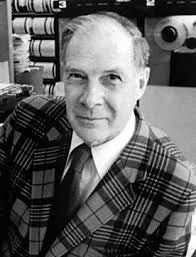Photo of Richard Hamming (1915-1998). Courtesy of Wikipedia.

Very briefly, the idea is to see how starting with one string, using a minimum number of insertions, deletions or substitutions it can be transformed into another string. From this point of view the string WORD is distance 2 from the string SWARD by using one substitution, O to A, and the insertion of an S. This approach to distance is one way to construct spell checking software for a word processor on a computer. If a word is typed that is not in the dictionary of words stored on the computer, perhaps the string which was typed is not really a word, so one tries to find a word in the dictionary that the data enterer might have meant by locating one or more words that are close or nearby to the typed word, using some way to measure the distance between strings.

Here is a very small example to give you the flavor of what can be done. To send an image, the image would be divided into a certain number of cells, often called pixels. In the simplest setting, each pixel might be black or white and one might send an image of the letter H represented in a 3x3 grid as shown below, using the code 0 for white and 1 for black. The image would be sent as 101111101 (one binary digit for each pixel). One may use the 0's and 1's to reconstruct the image, top to bottom and left to right.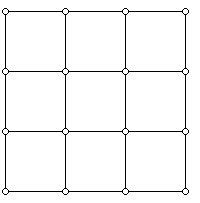A 3x3 pixel grid; grid used to represent the capital letter H with 9 black or white cells.

In recent times, a ubiquitous use of black and white cells in digital images is the QR (for Quick Response) code, a sample of which is displayed below.A QR digital code. Courtesy of Wikipedia.

When codes are used to represent information, it is traditional to use codes where the number of symbols in each code word is the same. This makes it possible to work in an environment where some kind of delimiter, such as a comma or a space, is not needed to separate code words. Thus, if 00 represents white and 11 represents black then one might send the message 00111100 rather than 00, 11, 11, 00.

It is worth remembering the way that using mathematics in the world and doing mathematics for its own sake reinforce each other. Mathematicians have been fascinated by cubes in many cultures and since ancient times. Their interest stemmed from the fact that they were regular polyhedra. Initially, cubes showed up in Euclid's Elements as one of the 5 convex solids in 3-space whose vertices are all alike and whose faces are (convex) regular polygons. Euclid does not mention the issue of convexity, a concept developed later by other mathematicians. These five polyhedra are now known as the Platonic Solids. But later mathematicians looked at the question of what regular convex solids existed in higher dimensions than 3. Intriguingly there are 6 regular convex solids in 4-dimensional space but in dimensions 5 or more there are only 3 regular convex polyhedra. But who might have realized that when the mathematics community studied the properties of cubes, they might be used in error correction technology?

Ancient scholars (and artists) interested in polyhedra did not have the modern tool of coordinates to use as a tool. However, eventually it was observed that the vertices of an $n$-dimensional cube could be labeled with all of the possible binary strings of length $n$. One can get between the labeled version of a $n$-cube and a ($n+1$)-cube in a delightfully appealing way that is illustrated below. What is shown is one way to represent a labeled 3-dimensional cube with its 8 vertices labeled with the 8 binary strings of length 3. Note that the bottom square in the diagram has all of its third coordinates 0 while the top square has all of its bottom coordinates 1. The 4 vertical edges join corresponding vertices in the two copies of the square (also known as a 2-cube) together. More generally, to get an $(n+1)$-cube from two copies of a labeled $n$-cube, in one copy of the $n$-cube one uses the binary sequences of length $n$ with a 0 added in the $n+1$ position while in the other copy of the $n$-cube one adds a 1 in the last position.A 3-dimensional cube labeled with the 8 binary strings of lengths 3

The 3-cube has 8 vertices and 12 edges. You can verify for yourself that the first diagram below can be thought of as a 4-dimensional cube with 16 vertices and 32 edges (as well as 24 square, two-dimensional faces). Cubes often give rise to attractive and symmetrical drawings. The first diagram below is appealing, but the fact that it represents a 4-dimensional cube is not so clear. The 5-cube and the 4-cubes which make it up can also be seen below. Based on the constructions shown, you can convince yourself that an $n$-cube has $2^n$ vertices and $n 2^{n-1}$ edges.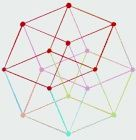A 4-cube diagram. Courtesy of Wikipedia.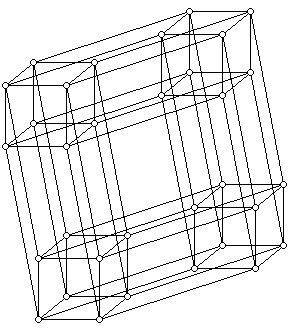A diagram representing the 5-cube, built up from two 4-cubes.

Given a binary string of length $n$, we can think of this string as a label for a vertex of an $n$-dimensional cube. The string we send conveys the information, for instance the gray level of a pixel in an image. If a string that is sent is not one of the possible strings in the dictionary whose entries consist of the binary digits in the code word, we know an error has been made, but typically this does not allow us to correct the string that arrived to the string that was sent. So the way we will conceptualize a code word is that some of its digits are information digits and that other digits are added with the goal of correcting errors.

If we want to code black or white pixels, we might use 0 to represent white and 1 to represent black, but if a 0 is sent a 1 might arrive and if a 1 is sent a 0 might arrive. A first idea might be that we could send the code word repeatedly, and though we are wasting space and time with sending a longer string than we need, perhaps this longer string will allow correction. Thus with the code 0, 1 we can sent 00 for 0 and 11 for 1. However, note that if 00 is sent and a single error occurs so that 10 arrives, we cannot be sure if this is because 00 was sent with an error or that 11 was sent and 10 arrived. In the first case an error occurred in position one and in the second case an error occurs in the second position. Thus, a single repetition is not good enough. We need something longer—000 or 111 would work, because now a single error allows us to use the notation of Hamming distance to tell which was the more likely sent string. For two strings of the same length, the Hamming distance between them is the number of positions in which the two strings differ. Thus, the strings 000 and 111 and the strings 101 and 110 have Hamming distance 3 and 2, respectively.

Major contributions to the theory of error correcting codes have arisen from practitioners of many backgrounds. I have already mentioned Richard Hamming but also remarkable are the contributions of Vera Pless(1931-2020) who made important contributions to coding theory as a researcher, teacher, and in applied settings.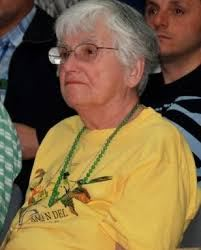Photo of Vera Pless. Courtesy of Wikipedia.

## Code transmission errors

Suppose one wants to code that the pixels in an image are black or white. Thus, one is trying to code two states or pieces of information. One would prefer to code this with short strings rather than longer ones. Using one bit will not work because if one codes black with 1 and white with zero, and a single bit is deleted what one receives is the empty message, and one cannot tell if a 0 or 1 was sent. If one uses 10 for black and 01 for white, then the received messages with one deletion are either 1 or 0. However if 1 arrives this can be due to the fact that 01 was sent and a 0 was deleted or if 10 was sent and a 0 was deleted. If one uses 00 and 11 as the code words, now if 0 arrives then 00 was sent while if 1 arrives 11 was sent and one can recover the original information.

$$00000, 10001, 01010, 11011, 11100, 00111$$

For each code word above, let us write down the result of one digit in the code word being deleted:

• 0000 (one string)
• 0001, 1001, 1000 (3 strings)
• 1010, 0010, 0110, 0100, 0101 (5 strings)
• 1011, 1111, 1101 (3 strings)
• 1100, 1110 (2 strings)
• 0111, 0011 (2 strings)

Notice that the number of possibilities can vary from one code word to another code word. In this example, since one deletion results in exactly 16 (1 + 3 + 5 + 3 + 2 + 2 = 16) possible binary sequences, this set of code words covers all of the possibilities and we can uniquely decide which string must have been sent for any choice of string that arrives.

When a set of code words is sent using hardware, it is possible that elements of the coded message may incur errors. Here are some ways that a communication channel might alter a sent code word:

• 10111 sent but 1111 arrived (though 5 symbols were sent only 4 were received)
• 10111 sent but 100111 arrived (though 5 symbols were sent 6 were received)
• 10111 was sent but 1?111 arrived (5 symbols were sent and 5 were received but the receiver is not sure what symbol was sent in the second position. It could be a 0 or 1, a reality indicated by using a ? to represent the fact that the receiver is not sure if a 0 or 1 arrived.
• 10111 was sent but 11111 arrived (5 symbols were sent and 5 received but there was an error in the second position; a 1 arrived though a 0 was sent in position 2 so the received string is 11111.

To design ways to digitally work with texts and images sent via a communications system, the notion of a mathematical channel was pioneered. The idea is that one transmits a sequence of data in the form of binary digits but what one receives at the other end of the channel may be different from what is transmitted in specific ways. Thus, in one model a 1 might change to a 0 with certain probability, or a zero might be changed to a 1 with the same probability p. This channel is probably the most studied and is known as the binary symmetric channel. Other kinds of communications channels can be described that relate to the kinds of errors that were briefly looked at above.

## Deletion error codes

The specific case that interests me is that of a communications channel where binary strings of the same length are used to encode information, and where, when a string is sent, it may be corrupted by a single deletion of one of the sent binary bits. Note that if one is expecting a code word, say, with 5 bits and only 4 bits arrive, one knows that a deletion error has occurred. It turns out that there is a strong relationship between the theory of codes involving deletion errors and insertion errors but only deletion error codes will be addressed here. In addition to Levenshtein, other pioneers of error deletion codes were Grigory Tenengolts and Rom Rubenovich Varshamov (1927-1929) a Soviet/Armenian mathematician).

So the general question to be studied is: If there are $k$ symbols, characteristics, etc. to be encoded, what is the largest number of binary code words of a fixed length $n$ that can used to capture the information one wants to transmit, when a code word might be subjected to the deletion of one binary bit? Above we saw that we could construct a binary code of length 5 with six code words which could correct one deletion error. You can verify that for strings of length 3, a code with two code words exists: 000, 101. Can you find a binary collection of code words of length 4 that will correct one error deletion? For strings of length 6 it is known that a code with ten code words exists, but it is not so easy to find such a code.

We have seen that the code 000 and 101 can be used to correct one deletion. So can the code 111 and 010, which arises from the first code by interchanging 1 and 0. You can check your understanding by considering the following question. If the set of binary strings $B$ of length $n$ allows one to use these strings to correct one deletion, will the set $B^*$ arising by interchanging the 0's and 1's in the strings of $B$ also give rise to a code which will enable one to correct one deletion error?

In using the error deletion codes discussed above, we have in essence imagined that we have a dictionary of the erroneous strings that arise and we correct the erroneous string using this dictionary. Study the idea of how one could correct the erroneous string by doing calculations on the erroneous string and based on these calculations, reconstruct the string that was sent.

Compared with other kinds of error correction codes, surprisingly little is known about error deletion codes. The discussion above can be extended to have the possibility of more than one deletion error (codes for when 2 error deletions might occur) or where the alphabet involves more than the two symbols 0 and 1. (For DNA, the alphabet uses 4 symbols.)

Enjoy thinking about and learning about using mathematics to study digital communications systems!

## References

Abramson, N., Information and Coding, McGraw Hill, New York, 1963.

Berlekamp, E., Algebraic Coding Theory, McGraw-Hill, New York, 1968.

Berlekamp, E., (ed.), Key Papers in the Development of Coding Theory, IEEE Press, New York, 1974.

Blahut, R., Theory and Practice of Error Control Codes, Addison-Wesley, Reading, l983.

Blake, I., Algebraic Coding Theory: History and Development, Dowden, Hutchinson and Ross, Stroudsburg, 1973.

Hamming, R., Coding and Information Theory, Prentice-Hall, Englewood Cliffs, 1980.

Hill, R., A First Course in Coding Theory, Oxford U. Press, Oxford, l986.

Hoffman, D. et al, Algebraic Coding Theory, Charles Babbage Research Centre, Winnipeg, 1987.

Lin, S., An Introduction to Error-Correcting Codes, Prentice-Hall, Englewood Cliffs, 1970.

MacWilliams, Florence Jessie, and Neil James Alexander Sloane. The Theory of Error-correcting Codes. North-Holland, Amsterdam, 1977.

Peterson, W. and E. Weldon, Error-correcting Codes, 2nd. ed., MIT Press, Cambridge, l972.

Pless, V., Introduction to the Theory of Error-Correcting Codes, Wiley, New York, 1982.

Shannon, C. and W. Weaver, The Mathematical Theory of Communication, U. of Illinois Press, Urbana, 1949.

Sloane, Neil, On single-deletion-correcting codes, Codes and designs, 10 (2000) 273-291.

Tatwawadi, Kedar, and Shubham Chandak, Tutorial on algebraic deletion correction codes, arXiv preprint arXiv:1906.07887 (2019).

Thompson, T., From Error-Correcting Codes Through Sphere Packing to Simple Groups, Mathematical Association of America, Washington, 1983.

Viterbi, A. and J. Omura, Principles of Digital Communication and Coding, Mc-Graw Hill, New York, 1979.

Welsh, D., Codes and Cryptography, Oxford U. Press, Oxford, 1988.

### 1 Comment

1.Great article Joe. Very clearly explained and the 4 and 5 dimensional “drawings” are amazing. Always loved the 5 Plutonic Solids and making models of them. Thanks for sharing.

Howard Brenner